# lrwpanWaveformGenerator

Generate low-rate wireless personal area waveform

Since R2023a

Download Required: To use `lrwpanWaveformGenerator`, first download the Communications Toolbox Library for ZigBee and UWB add-on.

## Syntax

``wave = lrwpanWaveformGenerator(data,cfg)``
``wave = lrwpanWaveformGenerator(data,cfg,Name=Value)``
``[wave,symbols] = lrwpanWaveformGenerator(___)``

## Description

example

````wave = lrwpanWaveformGenerator(data,cfg)` generates a waveform for the specified input, `data`, and physical layer (PHY) format configuration, `cfg`. For more information, see IEEE 802.15.4 PPDU Format.```

example

````wave = lrwpanWaveformGenerator(data,cfg,Name=Value)` specifies additional options using one or more name-value pair arguments. For example, `lrwpanWaveformGenerator(data,cfg,NumPackets=30)` specifies a waveform containing 30 PPDU packets.```
````[wave,symbols] = lrwpanWaveformGenerator(___)` also returns `symbols`, the HRP signal before pulse shaping. The `symbols` output contains the modulated signal after the preamble insertion. This syntax applies only when `cfg` is an `lrwpanHRPConfig` configuration object.```

## Examples

collapse all

Generate random data for a PHY Service Data Unit (PSDU) payload. Create an OQPSK IEEE 802.15.4 `lrwpanOQPSKConfig` object. Create an OQPSK IEEE 802.15.4 waveform.

``` psdu = randi([0,1],127*8,1); spc = 8; cfgOQPSK = lrwpanOQPSKConfig( ... SamplesPerChip=spc, ... PSDULength=length(psdu)/8); % PSDU length in bytes```

Generate an OQPSK IEEE 802.15.4 waveform by using the `lrwpanWaveformGenerator` function and `cfgOQPSK` configuration object. The waveform generator loops PSDU length as needed to use all input PSDU payload bits. Display the waveform by using the `eyediagram` function.

``` waveOQPSK = lrwpanWaveformGenerator(psdu,cfgOQPSK); eyediagram(waveOQPSK(1+spc:end-spc), 2*spc);```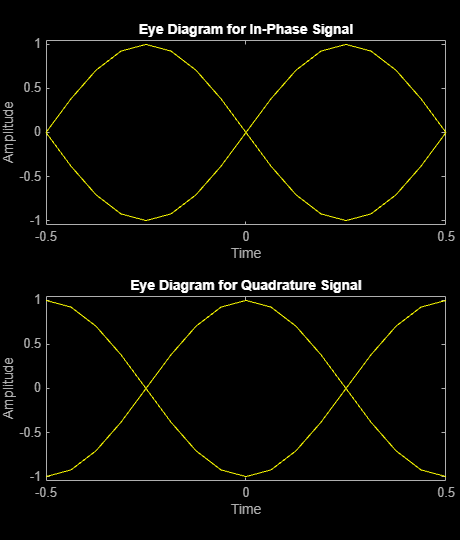Generate random data for a PHY Service Data Unit (PSDU) payload. Create an HPRF IEEE 802.15.4z `lrwpanHRPConfig` object.

```psdu = randi([0,1],8*200,1); cfgHPRF = lrwpanHRPConfig(Mode='HPRF', ... Channel=3, ... % Mandatory low-band channel MeanPRF=124.8, ... % 16 chips per payload symbol STSPacketConfiguration=1, ... % Enable STS before payload NumSTSSegments=2, ... % 2 STS segments CodeIndex=27, ... % One 91-symbols long SYNC code PreambleDuration=32, ... % Repetitions for spread SYNC code SFDNumber=1, ... % Choose a 4-symbol long SFD ConstraintLength=7, ... % Convolutional encoder, no RS coding PSDULength=100); % PSDU length in bytes```

Generate an HPRF IEEE 802.15.4z waveform by using the `lrwpanWaveformGenerator` function and `cfgHPRF` configuration object. The waveform generator loops PSDU length as needed to use all input PSDU payload bits. Display the waveform by using a `spectrumAnalyzer` object.

```waveHPRF = lrwpanWaveformGenerator(psdu,cfgHPRF); sa = spectrumAnalyzer(SampleRate=cfgHPRF.SampleRate); sa(waveHPRF);```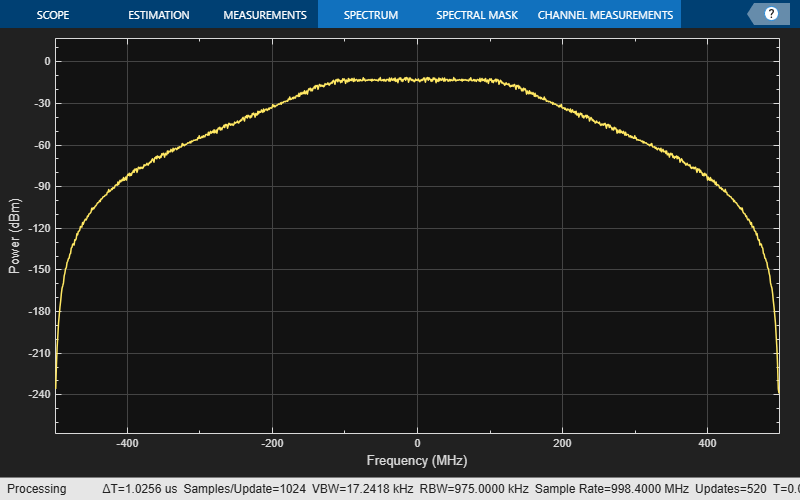Generate random data for a PHY Service Data Unit (PSDU) payload. Create a BPRF IEEE 802.15.4z `lrwpanHRPConfig` object. BPRF payload data rate is always at 6.81 Mbps.

```psdu = randi([0,1],8*100,1); cfgBPRF = lrwpanHRPConfig(Mode='BPRF', ... STSPacketConfiguration=0, ... % Turn off STS PHRDataRate=6.81, ... % PSDU header data rate in Mbps CodeIndex=9, ... % A 127-symbols long SYNC code PSDULength=length(psdu)/8); % PSDU length in bytes```

Generate an BPRF IEEE 802.15.4z waveform by using the `lrwpanWaveformGenerator` function and `cfgBPRF` configuration object. The waveform generator loops PSDU length as needed to use all input PSDU payload bits. Display the waveform by using a `spectrumAnalyzer` object.

```waveBPRF = lrwpanWaveformGenerator(psdu,cfgBPRF); sa = spectrumAnalyzer(SampleRate=cfgBPRF.SampleRate); sa(waveBPRF);```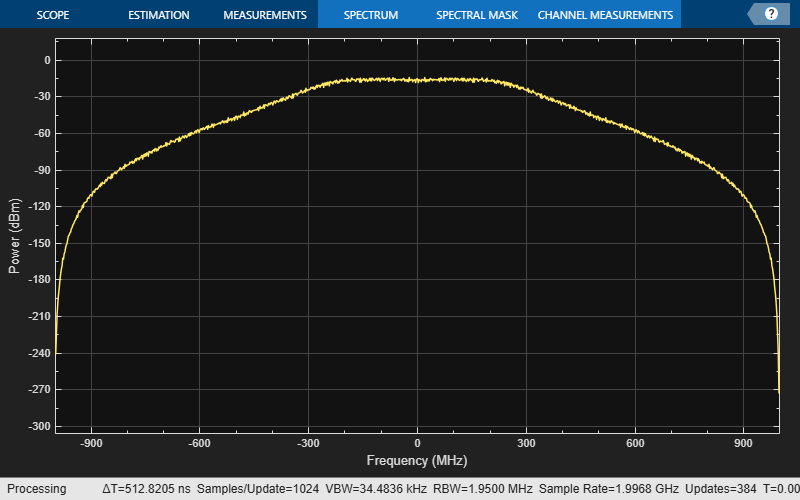Generate random data for a PHY Service Data Unit (PSDU) payload. Create an IEEE 802.15.4a `lrwpanHRPConfig` object.

```psdu = randi([0,1],50,1); cfg4a = lrwpanHRPConfig(Mode='802.15.4a', ... Channel=9, ... % High-band mandatory chan for code index 3 MeanPRF=15.6, ... % 8 candidate bursts DataRate=27.24, ... % 1 chip per burst (PHR at 850 kbps max) CodeIndex=3, ... % 3rd code with length 31 PreambleMeanPRF=4.03, ... % PreambleSpreadingFactor = 64 PSDULength=100); % PSDU length in bytes```

Generate an 4a IEEE 802.15.4z waveform by using the `lrwpanWaveformGenerator` function and `cfg4a` configuration object. The waveform generator loops input PSDU payload bits as needed to fill PSDU length. Display the waveform by using a `spectrumAnalyzer` object.

```wave4a = lrwpanWaveformGenerator(psdu,cfg4a); sa = spectrumAnalyzer(SampleRate=cfg4a.SampleRate); sa(wave4a);```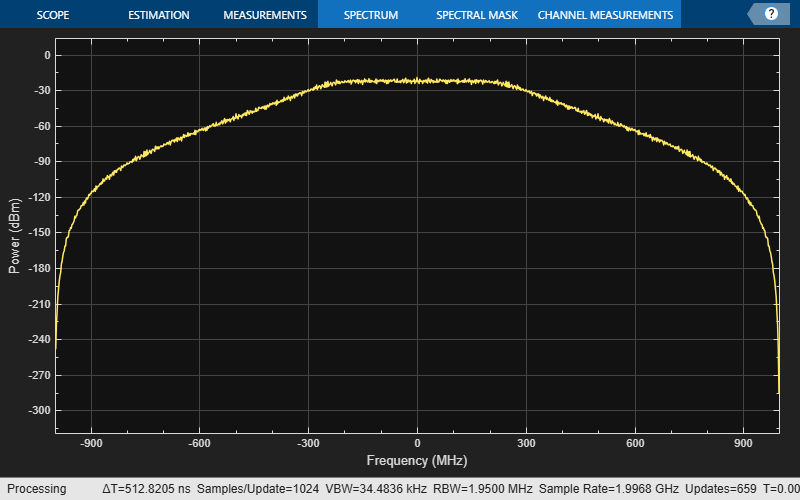Generate random data for a PHY Service Data Unit (PSDU) payload. Create a BPRF IEEE 802.15.4z `lrwpanHRPConfig` object. BPRF payload data rate is always at 6.81 Mbps.

```psdu = randi([0,1],8*100,1); cfgBPRF = lrwpanHRPConfig(Mode='BPRF', ... STSPacketConfiguration=0, ... % Turn off STS PHRDataRate=6.81, ... % PSDU header data rate in Mbps CodeIndex=9, ... % A 127-symbols long SYNC code PSDULength=length(psdu)/8); % PSDU length in bytes```

Generate a multiframe BPRF IEEE 802.15.4z waveform by using the `lrwpanWaveformGenerator` function and `cfgBPRF` configuration object. The waveform generator loops PSDU length as needed to use all input PSDU payload bits. Display the waveform by using a `timescope` object.

```waveBPRF = lrwpanWaveformGenerator(psdu,cfgBPRF, ... NumPackets=3, ... IdleTime=1e-4); ts = timescope(SampleRate=cfgBPRF.SampleRate, ... TimeSpanSource="property", ... TimeSpan=length(waveBPRF)/cfgBPRF.SampleRate, ... YLimits=1.1*[min(waveBPRF), max(waveBPRF)]); ts(waveBPRF);```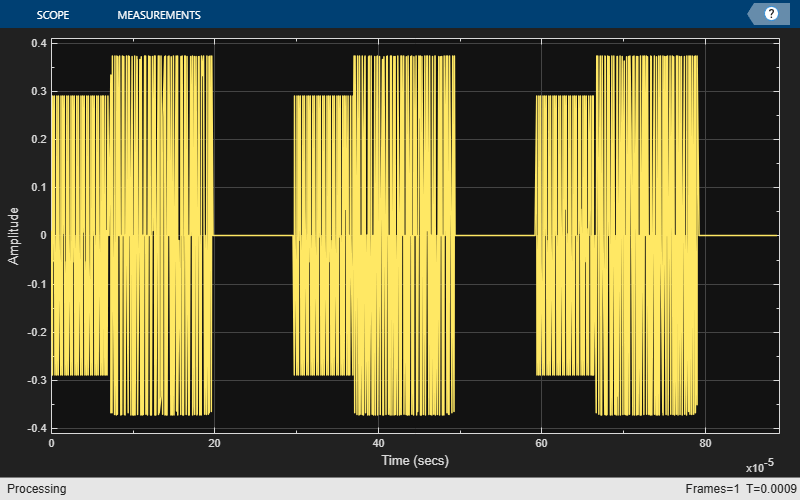## Input Arguments

collapse all

Information bits, specified as a binary-valued column vector. Each transmission packet uses (`cfg`.`PSDULength`×`8`) bits from `data`. The function loops on `data` as needed to generate the requested number of packets, `NumPackets`. If the number of bits required for all generated packets exceeds the length of `data`, the `data` vector is looped as needed to generate the requested waveform. Looping on the bits allows you to define a short pattern, such as `[1; 0; 0; 1]`, that repeats as the input to the PSDU coding across packets.

Data Types: `double` | `int8`
Complex Number Support: Yes

Packet format configuration, specified as an `lrwpanHRPConfig` or `lrwpanOQPSKConfig` object. The type of object you specify determines the IEEE® 802.15.4™ format of the output waveform, `wave`. The properties of the packet format configuration object determine the data rate and PSDU length of generated PPDUs.

### Name-Value Arguments

Specify optional pairs of arguments as `Name1=Value1,...,NameN=ValueN`, where `Name` is the argument name and `Value` is the corresponding value. Name-value arguments must appear after other arguments, but the order of the pairs does not matter.

Before R2021a, use commas to separate each name and value, and enclose `Name` in quotes.

Example: `NumPackets=21,IdleTime=15e-6`

Number of packets to generate in a single function call, specified as a positive integer.

Idle time added after each packet in seconds, specified as a nonnegative scalar.

## Output Arguments

collapse all

Packetized waveform, returned as a column vector. `wave` contains one or more packets of the same PPDU format. Each packet can contain different information bits. For more information, see IEEE 802.15.4 PPDU Format.

Data Types: `double`
Complex Number Support: Yes

HRP signal before pulse shaping, returned as a column vector of ternary values, specifically [`-1`, `0`, `1`]. `symbols` contains the modulated (symbol mapped) signal after preamble insertion.

#### Dependencies

This output applies when the input configuration object, `cfg`, is an `lrwpanHRPConfig` object.

Data Types: `double`
Complex Number Support: Yes

collapse all

### IEEE 802.15.4 PPDU Format

The IEEE standards for low-rate wireless networks,  and , specify IEEE 802.15.4 PHY protocol data unit (PPDU) formats for transmission of O-QPSK PHY and HRP UWB PHY waveforms. For all formats, the PPDU field structure includes preamble and payload portions. This figure shows the PPDU headers and payloads as defined in Figure 5-7 of .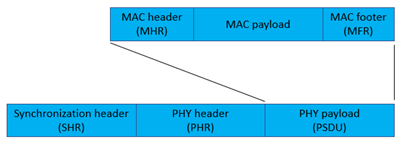• The `lrwpanHRPConfig` object specifies configuration for the HRP UWB PPDU format — The output waveform is a high-rate pulse repetition frequency (HRP) ultra-wide band (UWB) IEEE 802.15.4 a/z waveform. If the `Mode` property of the object is:

• `'HPRF'` — The output is a higher pulse repetition frequency (HPRF) waveform.

• `'BPRF'` — The output is a base pulse repetition frequency (BPRF) waveform.

• `'802.15.4a'` — The output is an IEEE 802.15.4a™ waveform.

• The `lrwpanOQPSKConfig` object specifies configuration for the O-QPSK PPDU format — The output waveform is a ZigBee® O-QPSK IEEE 802.15.4 waveform.

### Waveform Looping

To produce a continuous input stream, you can have your code loop on a waveform from the last packet back to the first packet.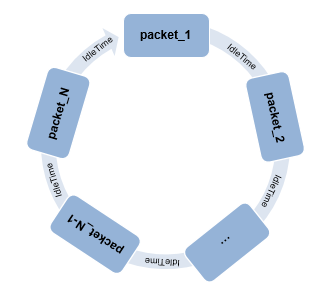When looping a waveform, the last symbol of packet_N is followed by the first symbol of packet_1. If the waveform has only one packet, the waveform loops from the last symbol of the packet to the first symbol of the same packet.

Specify the idle time by using the `IdleTime` input to the `lrwpanWaveformGenerator` function.

 IEEE STD 802.15.4-2020, IEEE Standard for Low-Rate Wireless Networks. May 2020.

 IEEE STD 802.15.4z-2020: IEEE Standard for Low-Rate Wireless Networks. Amendment 1: Enhanced Ultra Wideband (UWB) Physical Layers (PHYs) and Associated Ranging Techniques. June 2020.